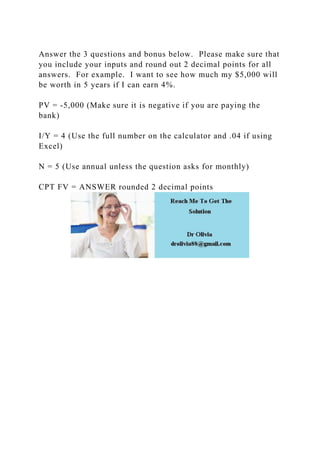Successfully reported this slideshow.

# Answer the 3 questions and bonus below.  Please make sure that you i.docx×

# Answer the 3 questions and bonus below.  Please make sure that you i.docx

Answer the 3 questions and bonus below.  Please make sure that you include your inputs and round out 2 decimal points for all answers.  For example.  I want to see how much my \$5,000 will be worth in 5 years if I can earn 4%.

PV = -5,000 (Make sure it is negative if you are paying the bank)

I/Y = 4 (Use the full number on the calculator and .04 if using Excel)

N = 5 (Use annual unless the question asks for monthly)

CPT FV = ANSWER rounded 2 decimal points
.

Answer the 3 questions and bonus below.  Please make sure that you include your inputs and round out 2 decimal points for all answers.  For example.  I want to see how much my \$5,000 will be worth in 5 years if I can earn 4%.

PV = -5,000 (Make sure it is negative if you are paying the bank)

I/Y = 4 (Use the full number on the calculator and .04 if using Excel)

N = 5 (Use annual unless the question asks for monthly)

CPT FV = ANSWER rounded 2 decimal points
.